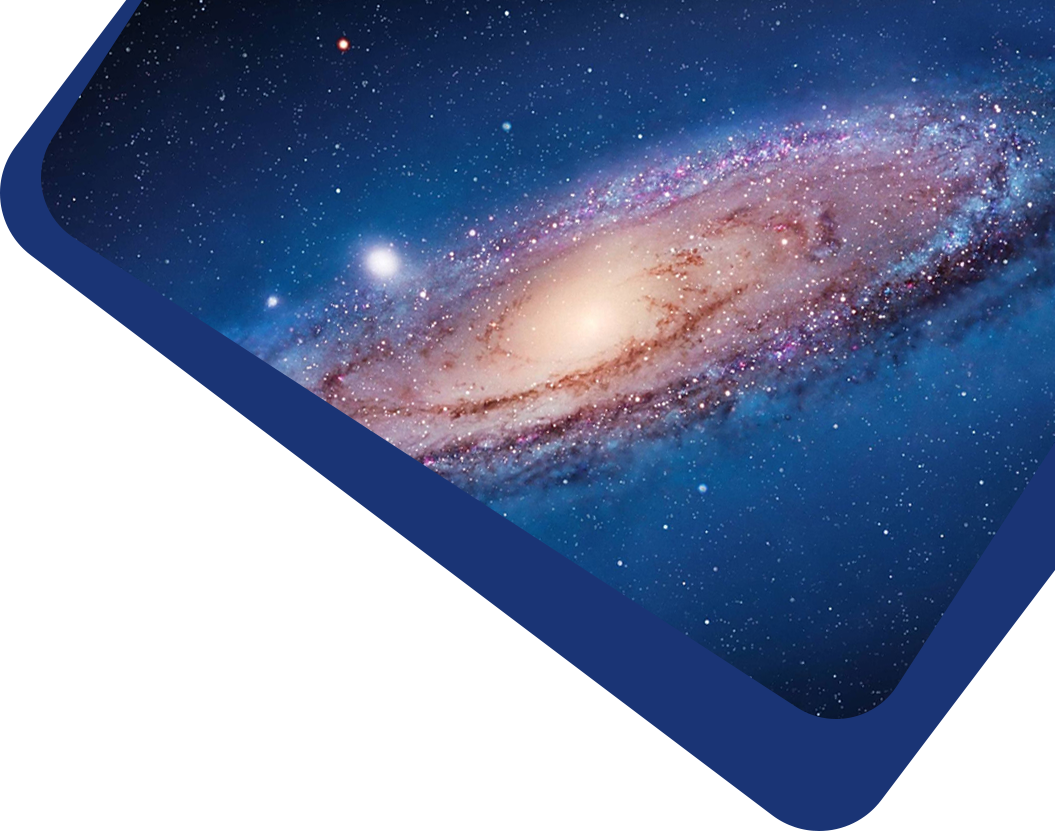Vol 22, No 12

## Torsion Fields Generated by the Quantum Effects of Macro-bodies

#### Da-Ming Chen

Abstract

We generalize Einstein's General Relativity (GR) by assuming that all matter (including macro-objects) has quantum effects. An appropriate theory to fulfill this task is Gauge Theory Gravity (GTG) developed by the Cambridge group. GTG is a "spin-torsion" theory, according to which, gravitational effects are described by a pair of gauge fields defined over a flat Minkowski background spacetime. The matter content is completely described by the Dirac spinor field, and the quantum effects of matter are identified as the spin tensor derived from the spinor field. The existence of the spin of matter results in the torsion field defined over spacetime. Torsion field plays the role of Bohmian quantum potential which turns out to be a kind of repulsive force as opposed to the gravitational potential which is attractive. The equivalence principle remains and essential in this theory so that GR is relegated to a locally approximate theory wherein the quantum effects (torsion) are negligible. As a toy model, we assume that the macro matter content can be described by the covariant Dirac equation and apply this theory to the simplest radially symmetric and static gravitational systems. Consequently, by virtue of the cosmological principle, we are led to a static universe model in which the Hubble redshifts arise from the torsion fields.

Keywords

cosmology: theory – (cosmology:) dark matter – (cosmology:) dark energy

Full Text
Refbacks